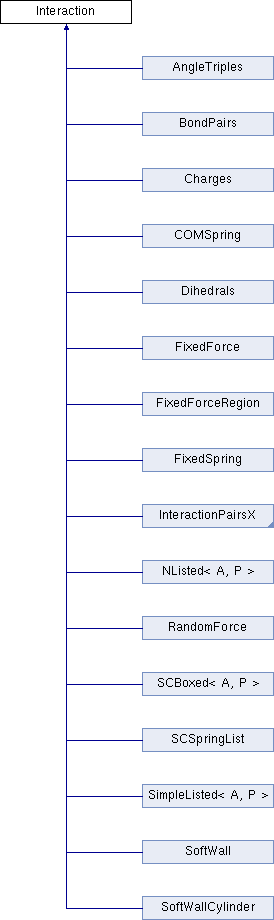ParM  parm A molecular dynamics library
Interaction Class Referenceabstract

The basic Interaction class, used to represent a potential function. More...

#include <interaction.hpp>

Inheritance diagram for Interaction:## Public Member Functions

virtual flt energy (Box &box)=0
Potential energy due to this Interaction. More...

virtual void set_forces (Box &box)=0

virtual flt set_forces_get_pressure (Box &box)
Set forces (Atom.f) and return $$P = \sum_{\left<i,j \right>} \vec r_{ij} \cdot \vec F_{ij}$$ at the same time (see pressure()). More...

virtual flt pressure (Box &box)=0
Partial pressure due to this Interaction. More...

virtual Matrix stress (Box &box)
The force-moment tensor for the current simulation: More...

virtual ~Interaction ()

## Detailed Description

The basic Interaction class, used to represent a potential function.

Specific interactions should derive from this.

## Constructor & Destructor Documentation

 virtual Interaction::~Interaction ( )
inlinevirtual

## Member Function Documentation

 virtual flt Interaction::energy ( Box & box )
pure virtual

Potential energy due to this Interaction.

 virtual flt Interaction::pressure ( Box & box )
pure virtual

Partial pressure due to this Interaction.

$$P = \sum_{\left<i,j \right>} \vec r_{ij} \cdot \vec F_{ij}$$, or equivalently $$P = \sum_i \vec r_i \cdot \vec F_i$$

Note that the full pressure involves all interactions and temperature, and needs to be normalized by $$\frac{1}{dV}$$ where $$d$$ is the number of dimensions and $$V$$ is the volume.

 virtual void Interaction::set_forces ( Box & box )
pure virtual
 virtual flt Interaction::set_forces_get_pressure ( Box & box )
inlinevirtual

Set forces (Atom.f) and return $$P = \sum_{\left<i,j \right>} \vec r_{ij} \cdot \vec F_{ij}$$ at the same time (see pressure()).

 virtual Matrix Interaction::stress ( Box & box )
inlinevirtual

The force-moment tensor for the current simulation:

$$\tau^{\alpha \beta} = \sum_{\left<i,j \right>} r_{ij}^\alpha F_{ij}^\beta$$.

At $$T=0$$, the stress tensor $$\Sigma = \frac{1}{dV} \tau$$.

Reimplemented in SCSpringList, and NListed< A, P >.

The documentation for this class was generated from the following file: